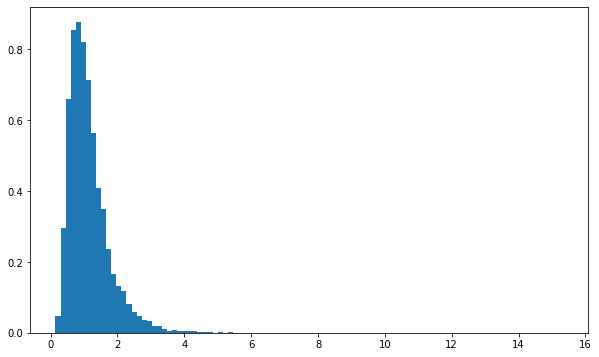Packages

import tensorflow as tf
import tensorflow_probability as tfp

import numpy as np
import matplotlib.pyplot as plt

tfd = tfp.distributions
tfpl = tfp.layers
tfb = tfp.bijectors

plt.rcParams['figure.figsize'] = (10, 6)
print("Tensorflow Version: ", tf.__version__)
print("Tensorflow Probability Version: ", tfp.__version__)
Tensorflow Version:  2.5.0
Tensorflow Probability Version:  0.13.0

Overview

The transformedDistribution is sort of distribution that can be defined by another base distribution and a bijector object. Tensorflow Probability offers transformed distribution object with consistent API that can use same methods and properties of other distribution.

normal = tfd.Normal(loc=0., scale=1.)
z = normal.sample(3)
z
<tf.Tensor: shape=(3,), dtype=float32, numpy=array([-2.270398  ,  0.18898423, -0.73362327], dtype=float32)>
scale_and_shift = tfb.Chain([tfb.Shift(1.), tfb.Scale(2.)])
x = scale_and_shift.forward(z)
x
<tf.Tensor: shape=(3,), dtype=float32, numpy=array([-3.5407958 ,  1.3779684 , -0.46724653], dtype=float32)>
log_prob_z = normal.log_prob(z)
log_prob_z
<tf.Tensor: shape=(3,), dtype=float32, numpy=array([-3.4962916, -0.936796 , -1.18804  ], dtype=float32)>
log_prob_x = (log_prob_z - scale_and_shift.forward_log_det_jacobian(z, event_ndims=0))
log_prob_x
<tf.Tensor: shape=(3,), dtype=float32, numpy=array([-4.189439 , -1.6299431, -1.8811872], dtype=float32)>

Note that, the event_ndims argument means the number of rightmost dimensions of z make up the event shape. So in the above case, the log of the jacobian determinant is calculated for each element of the tensor z.

Or we express it with the inverse of the bijective transformation.

log_prob_x = (log_prob_z + scale_and_shift.inverse_log_det_jacobian(x, event_ndims=0))
log_prob_x
<tf.Tensor: shape=(3,), dtype=float32, numpy=array([-4.189439 , -1.6299431, -1.8811872], dtype=float32)>

The result is the same as while using inverse of x.

log_prob_x = (normal.log_prob(scale_and_shift.inverse(x)) + scale_and_shift.inverse_log_det_jacobian(x, event_ndims=0))
log_prob_x
<tf.Tensor: shape=(3,), dtype=float32, numpy=array([-4.189439 , -1.6299431, -1.8811872], dtype=float32)>

You may notice that log probability of x can be calculated with only using z or x. In practice, most of cases uses second expression. The reason is that the z is from base distriubtion. So in terms of analysis, it is the latent variable. But x is from the data distribution, and it is the output from transformed distribution. While using mentioned approach, we can express transform object with bijector or invertible, it can be learned with best parameters for maximum likelihood.

# Base distribution                  Transformation                    Data distribution
# z ~ P0                <=>               x = f(z)            <=>            x ~ P1

log_prob_x = (base_dist.log_prob(bijector.inverse(x)) + bijector.inverse_log_det_jacobian(x, event_ndims=0))

### Training

x_sample = bijector.forward(base_dist.sample())
normal = tfd.Normal(loc=0., scale=1.)
z = normal.sample(3)
z
<tf.Tensor: shape=(3,), dtype=float32, numpy=array([1.1660839 , 0.26298988, 0.80137837], dtype=float32)>
exp = tfb.Exp()
x = exp.forward(z)
x
<tf.Tensor: shape=(3,), dtype=float32, numpy=array([3.2093997, 1.3008136, 2.2286105], dtype=float32)>
log_normal = tfd.TransformedDistribution(normal, exp)
log_normal
<tfp.distributions.TransformedDistribution 'expNormal' batch_shape=[] event_shape=[] dtype=float32>

Above expression is same with like this,

log_normal = exp(normal)
log_normal
<tfp.distributions.TransformedDistribution 'expNormal' batch_shape=[] event_shape=[] dtype=float32>
log_normal.sample()
<tf.Tensor: shape=(), dtype=float32, numpy=1.4088374>
log_normal.log_prob(x)
<tf.Tensor: shape=(3,), dtype=float32, numpy=array([-2.7648983, -1.2165103, -2.0414205], dtype=float32)>

We can also define specific event_shape and batch_shape for transformedDistribtion.

normal = tfd.Normal(loc=0., scale=1.)
scale_tril = [[1., 0.], [1., 1.]]
scale = tfb.ScaleMatvecTriL(scale_tril=scale_tril)
mvn = tfd.TransformedDistribution(tfd.Sample(normal, sample_shape=), scale)
mvn
<tfp.distributions.TransformedDistribution 'scale_matvec_trilSampleNormal' batch_shape=[] event_shape= dtype=float32>
scale_tril = [[[1., 0.], [1., 1.]], [[0.5, 0.], [-1., 0.5]]]
scale = tfb.ScaleMatvecTriL(scale_tril=scale_tril)

mvn = tfd.TransformedDistribution(tfd.Sample(tfd.Normal(loc=[0., 0.], scale=1.), sample_shape=, ), scale)
mvn
<tfp.distributions.TransformedDistribution 'scale_matvec_trilSampleNormal' batch_shape= event_shape= dtype=float32>

Tutorial

TransformedDistribution

n = 10000
loc = 0
scale = 0.5
normal = tfd.Normal(loc=loc, scale=scale)
print('batch shape: ', normal.batch_shape)
print('event shape: ', normal.event_shape)
batch shape:  ()
event shape:  ()
exp = tfb.Exp()
log_normal_td = exp(normal)
print('batch shape: ', log_normal_td.batch_shape)
print('event shape: ', log_normal_td.event_shape)
batch shape:  ()
event shape:  ()
z = normal.sample(n)

Plots

plt.hist(z.numpy(), bins=100, density=True)
plt.show()x = log_normal_td.sample(n)
plt.hist(x.numpy(), bins=100, density=True)
plt.show()log_normal = tfd.LogNormal(loc=loc, scale=scale)
l = log_normal.sample(n)
plt.hist(l.numpy(), bins=100, density=True)
plt.show()Log probability

log_prob = log_normal.log_prob(x)
log_prob_td = log_normal_td.log_prob(x)
tf.norm(log_prob - log_prob_td)
<tf.Tensor: shape=(), dtype=float32, numpy=0.0>

Event shape and batch shape

tril = tf.random.normal((2, 4, 4))
scale_low_tri = tf.linalg.LinearOperatorLowerTriangular(tril)
scale_low_tri.to_dense()
<tf.Tensor: shape=(2, 4, 4), dtype=float32, numpy=
array([[[-0.71624756,  0.        ,  0.        ,  0.        ],
[ 0.5268538 , -1.0188125 ,  0.        ,  0.        ],
[ 0.76072276, -0.5344736 ,  0.4067452 ,  0.        ],
[ 0.43506932, -0.8857401 , -1.8538325 ,  1.9112511 ]],

[[-1.3100191 ,  0.        ,  0.        ,  0.        ],
[ 0.6303728 ,  0.42918292,  0.        ,  0.        ],
[ 1.1252751 , -0.66470486, -0.71725285,  0.        ],
[ 2.2047887 , -0.48715273, -0.694466  , -1.1677382 ]]],
dtype=float32)>
scale_lin_op = tfb.ScaleMatvecLinearOperator(scale_low_tri)
mvn = tfd.TransformedDistribution(tfd.Sample(tfd.Normal(loc=[0., 0.], scale=1.), sample_shape=), scale_lin_op)
print('batch shape: ', mvn.batch_shape)
print('event shape: ', mvn.event_shape)
batch shape:  (2,)
event shape:  (4,)
y1 = mvn.sample(sample_shape=(n,))
print(y1.shape)
(10000, 2, 4)
mvn2 = tfd.MultivariateNormalLinearOperator(loc=0, scale=scale_low_tri)
mvn2
<tfp.distributions.MultivariateNormalLinearOperator 'MultivariateNormalLinearOperator' batch_shape= event_shape= dtype=float32>
y2 = mvn2.sample(sample_shape=(n, ))
y2.shape
TensorShape([10000, 2, 4])
xn = normal.sample((n, 2, 4))
tf.norm(mvn.log_prob(xn) - mvn2.log_prob(xn)) / tf.norm(mvn.log_prob(xn))
<tf.Tensor: shape=(), dtype=float32, numpy=0.0>